﻿ 江西省对外贸易与经济增长效应分析

# 江西省对外贸易与经济增长效应分析Analysis of the Effect of Foreign Trade and Economic Growth in Jiangxi Province

Abstract: Foreign trade is an important indicator of economic growth to measure regional economic development and an inherent requirement of economic development. Based on the VAR model, this paper empirically analyzes the import growth rate, export growth rate and Jiangxi provincial GDP growth rate by using the data of Jiangxi Province from 1995 to 2018. The results show that the three variables have a long-term equilibrium relationship. The economic growth of Jiangxi Province has a positive effect on both export trade and import trade, especially for import trade. The pulling effect of import trade on economic growth will be weakened year by year, and the effect of export trade on economic growth will be more significant in the long run.

1. 引言

2. 文献综述

3. 江西省经济增长与对外贸易概况

3.1. 江西省经济增长现状

3.2. 江西省对外贸易现状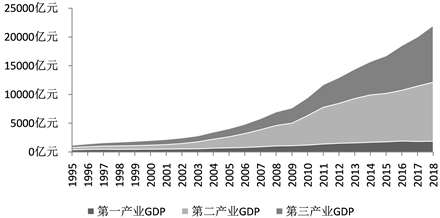数据来源：Wind数据库整理所得。

Figure 1. Schematic diagram of three industrial output values in Jiangxi province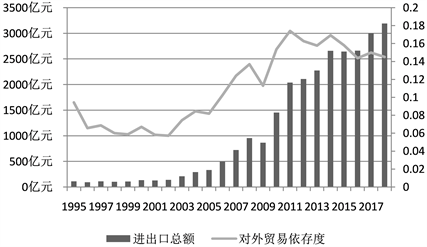数据来源：Wind数据库整理所得。

Figure 2. Schematic diagram of the changes in the total foreign trade and foreign trade dependence of Jiangxi province

4. 数据选取及变量检验

4.1. 模型和方法

VAR模型常用来表示多变量之间的动态关系，不依赖于任何约束条件，对当期值和若干滞后期进行回归。P阶VAR模型的基本表达式为：

${y}_{t}=\alpha +{\beta }_{0}{x}_{t-1}+{\beta }_{1}{x}_{t-2}+\cdot \cdot \cdot +{\beta }_{p}{x}_{t-p}+{\epsilon }_{t}$

4.2. 数据选取Table 1. Statistical description of variables

4.3. 变量平稳性检验Table 2. Variable stationarity test results

4.4. 格兰杰关系检验Table 3. Granger relationship test result

4.5. 模型滞后期的选取Table 4. Standards for model lag period selection

5. 实证分析

5.1. 构建VAR模型

VAR模型可以研究变量之间相互关系，通过模型估计结果，每个内生变量都可以通过其他所有内生变量的滞后值来拟合。根据前文滞后期数准则确定最佳滞后期数为1阶。本文构建了RIM、REX、和RGDP三维向量的VAR模型，VAR(1)模型如下：

$\left(\begin{array}{c}RIM\\ REX\\ RGDP\end{array}\right)=\left(\begin{array}{c}0.3621\\ 0.114\\ 0.0358\end{array}\right)+\left(\begin{array}{ccc}0.1789& 0.1288& -2.579\\ 0.2252& 0.0441& 0.4793\\ 0.0746& 0.0767& 0.0336\end{array}\right)\left(\begin{array}{c}RI{M}_{t-1}\\ RE{X}_{t-1}\\ RGD{P}_{t-1}\end{array}\right)+\left(\begin{array}{c}{\epsilon }_{1t}\\ {\epsilon }_{2t}\\ {\epsilon }_{3t}\end{array}\right)$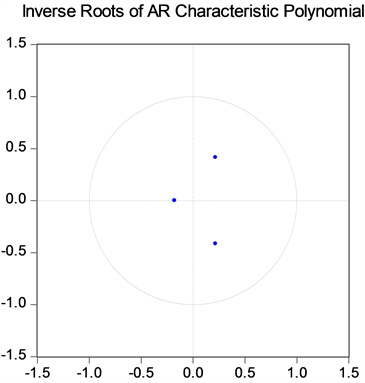Figure 3. VAR model stationarity test results

5.2. 脉冲响应分析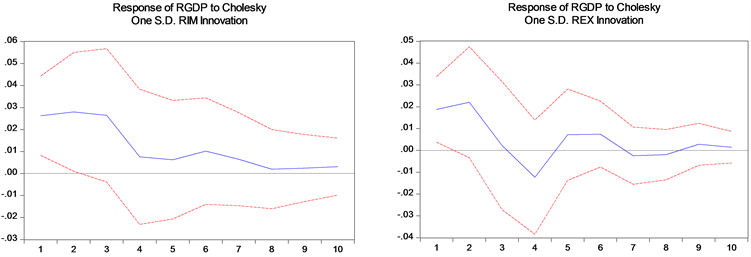Figure 4. Impulse response diagram of the response of RGDP to RIM and REX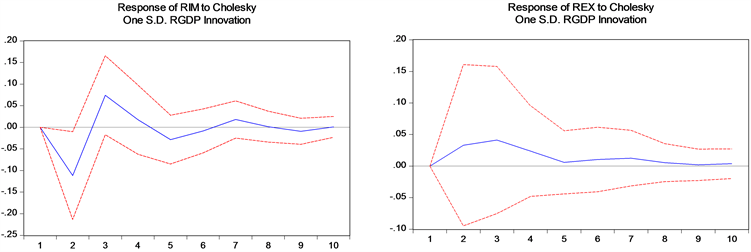Figure 5. Impulse response diagram of the response of RIM and REX to RGDP

5.3. 方差分解分析Table 5. Variable RIM variance decomposition resultsTable 6. Variable REX variance decomposition resultsTable 7. Variable RGDP variance decomposition results

6. 结论与建议

 赵清军, 王雅妮, 周毕芬. 福建省经济增长与进出口贸易关系的实证分析[J]. 石家庄铁道大学学报(社会科学版), 2018, 12(4): 9-14.

 余升国, 梁湄淇, 魏域谦. 外贸依存度与经济增长——基于中国西部地区的实证分析[J]. 中外企业家, 2017(20): 6-7.

 刘爱珍, 漆雁斌. 基于VAR模型的我国对外贸易与经济增长关系实证研究[J]. 经济论坛, 2018, 578(9): 140-145.

 邢胜龙. 基于柯布-道格拉斯生产模型下的对外开放与区域经济增长关系研究[J]. 商业经济研究, 2019(8): 140-143.

 周红云, 李琦. 对外贸易对中国台湾经济增长有效性的影响研究——基于脉冲响应函数的实证分析[J]. 知识经济, 2019, 487(2): 68-69.

 窦旺胜, 王成新. 山东省对外贸易与经济增长关系研究[J]. 价格月刊, 2019(5): 28-34.

 曾珍. 对外贸易依存度及其应用研究[J]. 现代经济信息, 2018(20): 121.

Top